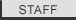Tabular Data Analysis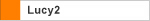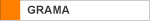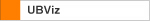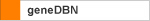Installing Perl Download Reference Manual Introduction Tutorials Numerical Data Extraction Statistical Data Extraction Patent Calculation PDB Data Extraction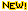GenBank Data Extraction Tabular Data AnalysisWord MappingDNA to Protein Extraction Change Log FAQ Cookbook
 Important: Before reading this tutorial you should already read the tutorial “Numerical Data Extraction”, since this tutorial uses several rules created in that tutorial, and you are assumed to be familiar with the procedure of adding your own perl program under Vect so that we can simply let this tutorial begin with more interesting things. OK, So you have read “Numerical Data Extraction” and know how to extract columns from the original table, and sum up two columns. Now let’s get a little bit more involved and do some more meaningful (and analytical) data extractions. Let’s use the same data file we were using in the tutorial “Numerical Data Extraction”. Suppose you are a consumer’s data analyst, and you were asked: “How many states have cigarette sales more than 100, and what are these states?” With Vect, a couple of lines of Perl code can solve this problem. First, you should select the data in the columns “State” and “Sales”, and make them numbers-only. You may follow the steps in Section 2 and Section 3 in the tutorial “Numerical Data Extraction” to do this. After those steps your Convert Data Panel should be similar to this one: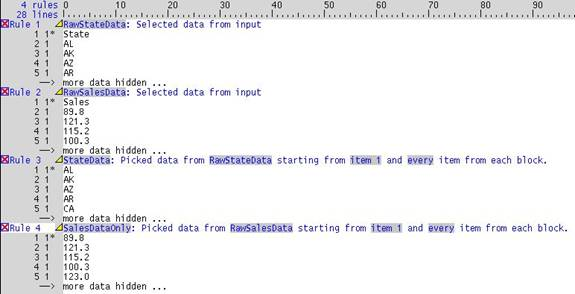Last modified June 13, 2008 . All rights reserved. Contact Webmaster